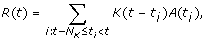Volume 10 Supplement 1

# Computing the inverse of the neurophysiological spike-response transform

Consider the transform from a discrete spike train to a continuous neurophysiological response such as postsynaptic membrane voltage or muscle contraction. Here we ask about the inverse of this transform: given the response, how can we estimate from it the spike train that produced it? A simple kernel-based model of such a transform is(1)

where t is time (already discretized for practical computation, so that t is the index of a series of time bins), t i is the time of spike i, K is the single-spike response kernel of length N K time bins, A is an amplitude that scales K at each spike time, and R is the overall response to the spike train. In previous work [1, 2], we developed a method to "decode" (1) to find, given the spike times and the response R, simultaneously both K and A. If the spike train is unknown, a spike might hypothetically occur in each time bin t'. In that case, (1) generalizes to the standard convolution(2)

The inverse transform can then be computed using (1) or (2), depending on the circumstances, in at least two ways. If, perhaps from a previous decoding of (1) with a known spike train, we know K, we can use our decoding method, or another standard method, to solve (2) in one step to find from a novel R(t) the corresponding A(t). In datasets with relatively low noise and low spike density, the spikes can then be identified simply as occurring in time bins where A(t) ≠ 0 or, in practice, where A(t) exceeds some threshold. Otherwise, A(t) still reflects the input presented by the spike train, albeit in a more diffuse way. A more challenging problem occurs if K is unknown. Then we can decode (1) to find K and A as previously [1, 2], except iteratively, starting with the assumption that each time bin contains a spike and progressively deleting spikes from those bins that have the smallest values of A. Figure 1 shows an example with synthetic data where this method simultaneously found K and A, reconstructed R, and identified each spike in the train, perfectly. We have used these methods successfully with synthetic data and with the real transforms from motor neuron spikes to postsynaptic membrane voltage and muscle contractions in the cardiac system of the blue crab .

## References

1. Stern E, Fort TJ, Miller MW, Peskin CS, Brezina V: Decoding neurophysiological responses to arbitrary spike trains. Soc Neurosci Abstr. 2006, 491.7.

2. Stern E, Fort TJ, Miller MW, Peskin CS, Brezina V: Decoding modulation of the neuromuscular transform. Neurocomputing. 2007, 70: 1753-1758. 10.1016/j.neucom.2006.10.117.

3. Stern E, Fort TJ, Miller MW, Peskin CS, Brezina V: Characterization of the crab cardiac neuromuscular transform. Soc Neurosci Abstr. 2007, 536.1.

## Acknowledgements

Supported by NIH grants NS058017, NS41497, GM08224, and RR03051.

Authors

## Rights and permissions

Reprints and Permissions